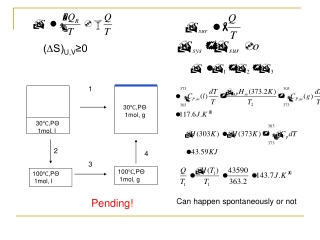DownloadDownload Presentation30℃,P Θ 1mol, l

30℃,P Θ 1mol, l

Télécharger la présentation30℃,P Θ 1mol, l

- - - - - - - - - - - - - - - - - - - - - - - - - - - E N D - - - - - - - - - - - - - - - - - - - - - - - - - - -
Presentation Transcript

1. 1 2 4 3 100℃,PΘ 1mol, g 100℃,PΘ 1mol, l 30℃,PΘ 1mol, g 30℃,PΘ 1mol, l Can happen spontaneously or not (∆S)U,V≥0 Pending!

2. Helmholtz free energies work function Helmholtz Justification 3.8 Helmholtz / Gibbs energies and their applications =, equilibrium(reversible); <, spontaneous

3. Remark Reversible phase shift ΔPTG=0 Gibbs free energy Gibbs justification Gibbs energies =, equilibrium(reversible); <, spontaneous

4. Understanding about A and G State function Variation driving force Energy---Work function

5. ΔH<<0 ΔS>>0 ΔG<0 ΔH<0 ΔS>0 Calculation of ΔA and ΔG Due to Calculating work • A=U-TS G=H-TS Due to calculating ΔU,ΔH,ΔS relating Q, W, ΔU, ΔH, ΔS, ΔA, ΔG

6. C8H18(l)+25/2O2 8CO2(g)+9H2O Octane Application : Predict the available maximum work • CH4(g)+2O2 CO2(g)+2H2O Methane

7. H2O(S),268K, 101325Pa H2O(l),268K, 101325Pa H2O(l),273K, 101325Pa H2O(l),273K, 101325Pa • 1mol supercooled water get frozen at 268K and 101325 Pa, the Wf,max, Wmax?

8. Clausius Justification 100℃,PΘ 1mol, g 25℃,PΘ 1mol, g 100℃,PΘ 1mol, l 25℃,PΘ 1mol, l To vacuum free Gibbs Helmholtz Predict the reaction direction W=0 ΔG=8285J > 0

9. Z: H, S, A, G ….. U, H, S, A, G, CP, CV, T, P, V 3.9 Thermodynamic relationships • Ten functions All state functions Closed system, no phase transformation, no chemical reaction, no composition change, 2 independent parameters Characteristic functions ----Massieu

10. Wf=o The fundamental equations Definitions W’=0 Closed system

11. Related Coefficient equations State variation only

12. The Maxwell relations T P V S

13. =0 Circling relation equation Chain relations Inverse relations Other mathematical relations

14. Heat capacity relations

15. 3.10 Properties of the Gibbs energy G(T, P, V) State variation Phase transformation or reaction:AB: G = GB – GA

16. Homework: • Y: P76: 23, 25, P77: 28; P79: 33 P80:37 • A: P132-134: 5.11, 5.18, 5.31 • Preview: • A: 7.1-7.3 • Y:3.1-3.4 • Group discussion: • I: Approaches to decrease greenhouse gas and save energy. • II: Understanding about the orderly assembly phenomenon in nature( entropy principle) • III: Where would the world go? Introduction about the dissipative structure principle.